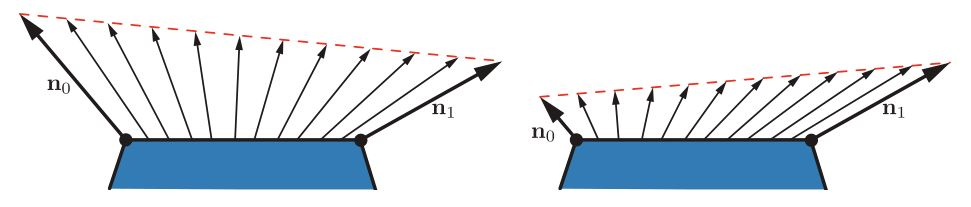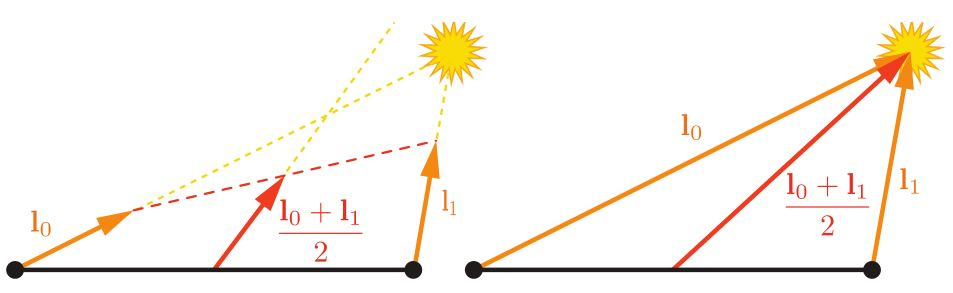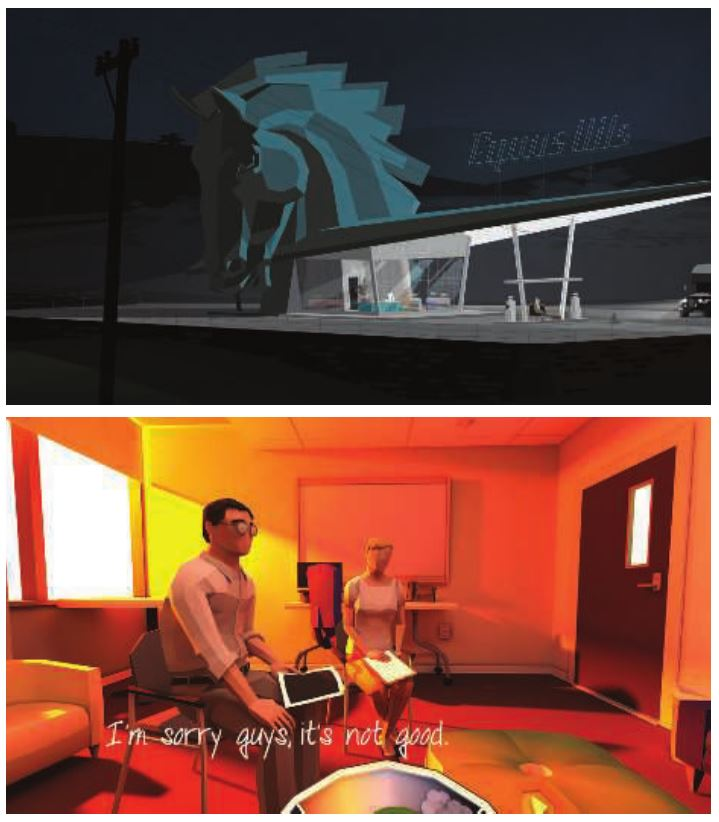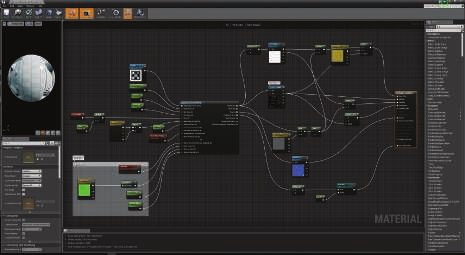# 第5章-着色基础-5.3-实现着色模型

## 5.3 实现着色模型

### 5.3.1 计算频率

• 顶点着色器——逐预细分顶点计算。
• 外壳着色器——逐表面片元计算。
• 域着色器——逐细分后顶点的计算。
• 几何着色器——逐图元计算。
• 像素着色器——逐像素计算。### 5.3.2 实现示例

$\textbf{c}_\textrm{shaded} = \frac{1}{2}\textbf{c}_\textrm{cool} + \sum^n_{i=1}(\textbf{l}_i \cdot \textbf{n})^{+}\textbf{c}_{\textrm{light}_i}(s_i\textbf{c}_\textrm{highlight} + (1 − s_i)\textbf{c}_\textrm{warm}) \tag{5.19}$

\begin{aligned} \textbf{c}_\textrm{cool} &= (0,0,0.55) + 0.25\textbf{c}_\textrm{surface} \\ \textbf{c}_\textrm{warm} &= (0.3,0.3,0) + 0.25\textbf{c}_\textrm{surface} \\ \textbf{c}_\textrm{highlight} &= (2,2,2) \\ \textbf{r}_i &= 2(\textbf{n} \cdot \textbf{l}_i)\textbf{n} − \textbf{l}_i \\ s_i &= (100(\textbf{r}_i \cdot \textbf{v}) − 97)^{\mp} \\ \end{aligned} \tag{5.20}

$\textbf{c}_\textrm{shaded} = f_\textrm{unlit}(\textbf{n},\textbf{v}) + \sum_{i = 1}^{n}({\textbf{l}_i}\cdot{\textbf{n}})^{+}\textbf{c}_{\textrm{light}_{i}}f_\textrm{lit}(\textbf{l}_{i},\textbf{n},\textbf{v})$

\begin{aligned} f_\textrm{unlit}(\textbf{n},\textbf{v}) &= \frac{1}{2}\textbf{c}_\textrm{cool} \\ f_\textrm{lit}(\textbf{l}_{i},\textbf{n},\textbf{v}) &= s_i\textbf{c}_\textrm{highlight} + (1 − s_i)\textbf{c}_\textrm{warm} \end{aligned} \tag{5.21}

in vec3 vPos;
in vec3 vNormal;
out vec4 outColor;


struct Light {
vec4 position;
vec4 color;
};

uniform LightUBlock {
Light uLights[MAXLIGHTS];
};

uniform uint uLightCount;


vec3 lit(vec3 l, vec3 n, vec3 v) {
vec3 r_l = reflect(-l, n);
float s = clamp(100.0 * dot(r_l, v) - 97.0, 0.0, 1.0);
vec3 highlightColor = vec3(2,2,2);
return mix(uWarmColor , highlightColor , s);
}

void main() {
vec3 n = normalize(vNormal);
vec3 v = normalize(uEyePosition.xyz - vPos);
outColor = vec4(uFUnlit , 1.0);

for (uint i = 0u; i < uLightCount; i++) {
vec3 l = normalize(uLights[i].position.xyz - vPos);
float NdL = clamp(dot(n, l), 0.0, 1.0);
outColor.rgb += NdL * uLights[i].color.rgb * lit(l,n,v);
}
}


layout(location=0) in vec4 position;
layout(location=1) in vec4 normal;
out vec3 vPos;
out vec3 vNormal;


void main() {
vec4 worldPosition = uModel * position;
vPos = worldPosition.xyz;
vNormal = (uModel * normal).xyz;
gl_Position = viewProj * worldPosition;
}


var fSource = document.getElementById("fragment").text.trim();

var maxLights = 10;
fSource = fSource.replace(/MAXLIGHTS/g, maxLights.toString());



### 5.3.3 材质系统

• 使用几何处理组合表面着色，例如刚性变换、顶点混合、变形、曲面细分、实例化和裁剪。这些功能位独立变化：表面着色取决于材质，几何处理取决于网格。因此，可以方便地分别编写它们并让材质系统根据需要组合它们。

• 使用合成操作（例如像素丢弃和混合）合成表面着色。这与移动GPU尤其相关，其中混合通常在像素着色器中执行。通常需要独立地用于表面着色的材质来选择这些操作。

• 将用于计算着色模型参数的操作与着色模型本身的计算组合起来。这允许创作着色模型实现一次，并结合各种不同的方法重新使用它来计算着色模型参数。

• 将可单独选择的材质特征相互组合、选择逻辑和着色器的其余部分。这使得分别编写每个功能的实现成为可能。

• 组合着色模型并计算其参数与光源计算：计算每个光源的着色点的$$\textbf{c}_\textrm{light}$$$$\textbf{l}$$值。诸如延迟渲染（第20章讨论）之类的技术改变了这种组合的结构。在支持多种此类技术的渲染框架中，这增加了额外的复杂性。

• 代码重用——在共享文件中实现函数，使用#include预处理器指令从需要它们的任何着色器访问这些函数。

• 加法——各种功能被定义为具有输入和输出连接器的节点，它们组合在一起。这类似于代码重用策略，但更加结构化。节点的组成可以通过文本或可视图形编辑器来完成。后者旨在使非工程师（例如技术艺术家）更容易创作新的材料模板[1750,1802]。通常只有部分着色器可供可视化图形创作使用。例如，在虚幻引擎中，图形编辑器只能影响着色模型输入的计算。请参见图5.13。

• 基于模板——定义了一个接口，不同的实现可以插入到该接口中，只要它们符合该接口。这比加法策略更正式一些，通常用于更大的功能块。这种接口的一个常见示例是着色模型参数的计算与着色模型本身的计算之间的分离。虚幻引擎具有不同的“材质域”，包括用于计算着色模型参数的表面域和用于计算为给定光源调制$$\textbf{c}_\textrm{light}$$的标量值的光函数域。Unity中也存在类似的“表面着色器”结构。请注意，延迟着色技术（在第20章中讨论）强制执行类似的结构，将G缓冲区用作接口。posted @ 2022-01-11 20:47  charlee44  阅读(313)  评论(0编辑  收藏  举报